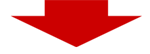## Calculate average speed online

How to use this average speed calculator: enter distance and time (days, hours, minutes, seconds) below. The distance can be entered in kilometers or miles as distance unit. Attn.: only use . (dot) as decimal separator!.

Enter distance and time:distance unit kilometermiles hours minuts seconds
 distance 0 total time (seconds) 0 average speed (m/s) nan average speed (km/h) nan average speed (miles/h) nan

#### Average Speed Calculation Formula

Simply divide the total distance by the time elapsed.